# NCERT Exemplar Class 11 Physics Solutions for Chapter 8 - Gravitation

NCERT exemplar class 11 physics Chapter 8 Gravitation is the foremost learning material that is very much essential for the students of CBSE class and for them who are preparing for engineering and medical entrance examinations. This NCERT exemplar class 11 physics Chapter 8 pdf has class 11 gravitation questions from NCERT exemplar books in addition to gravitation class 11 important derivations, solved numerical’s on gravitation, MCQ’S, worksheets, HOTS and exercises. Gravitation is simply a phenomenon that occurs in nature, where things with energy or mass are attracted to each other. These may be planets, stars and even galaxies. This force of attraction that exists between two objects causes them to gravitate towards each other. Albert Einstein initially proposed the general theory of relativity which described gravity most accurately.

## Download the PDF of NCERT Exemplar Class 11 Physics Chapter 8 Gravitation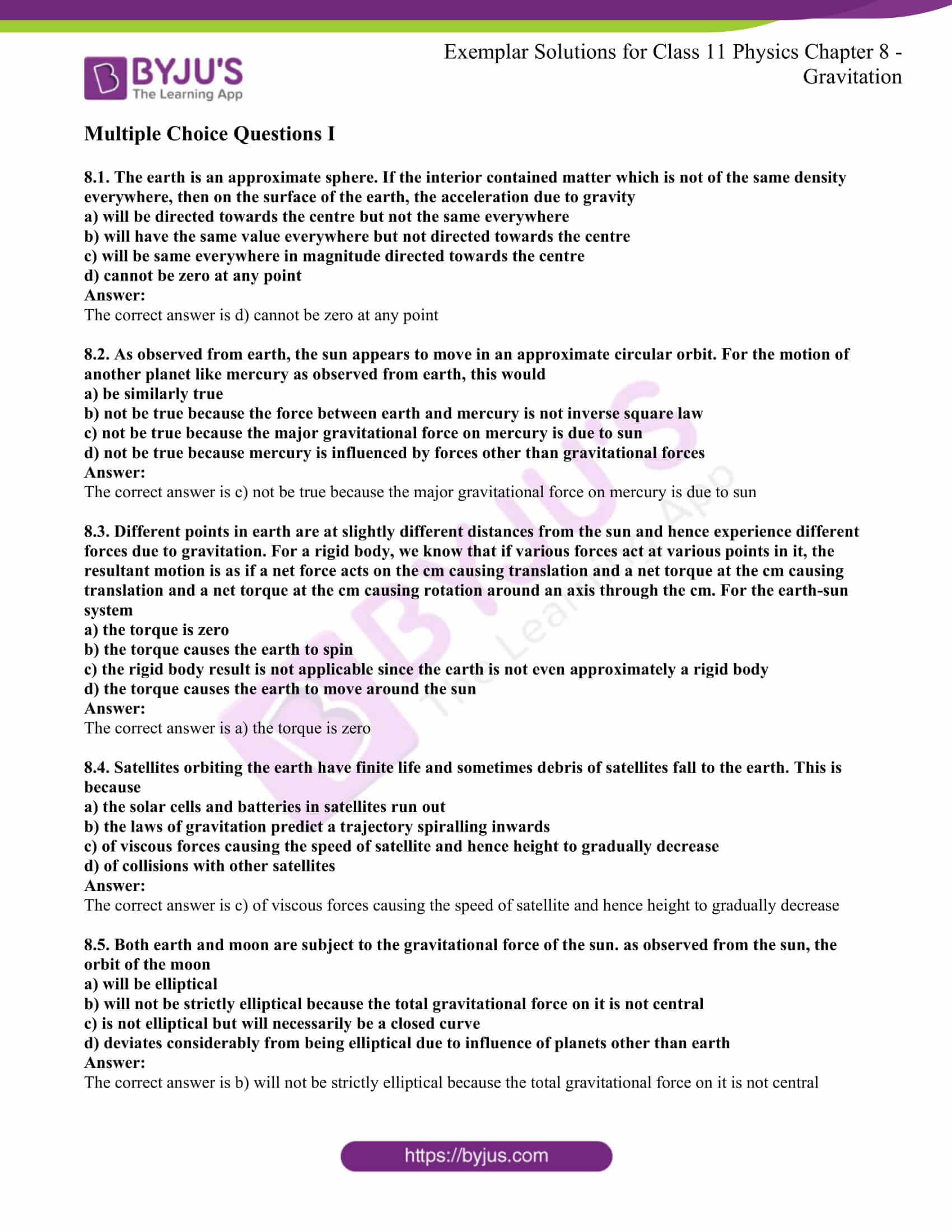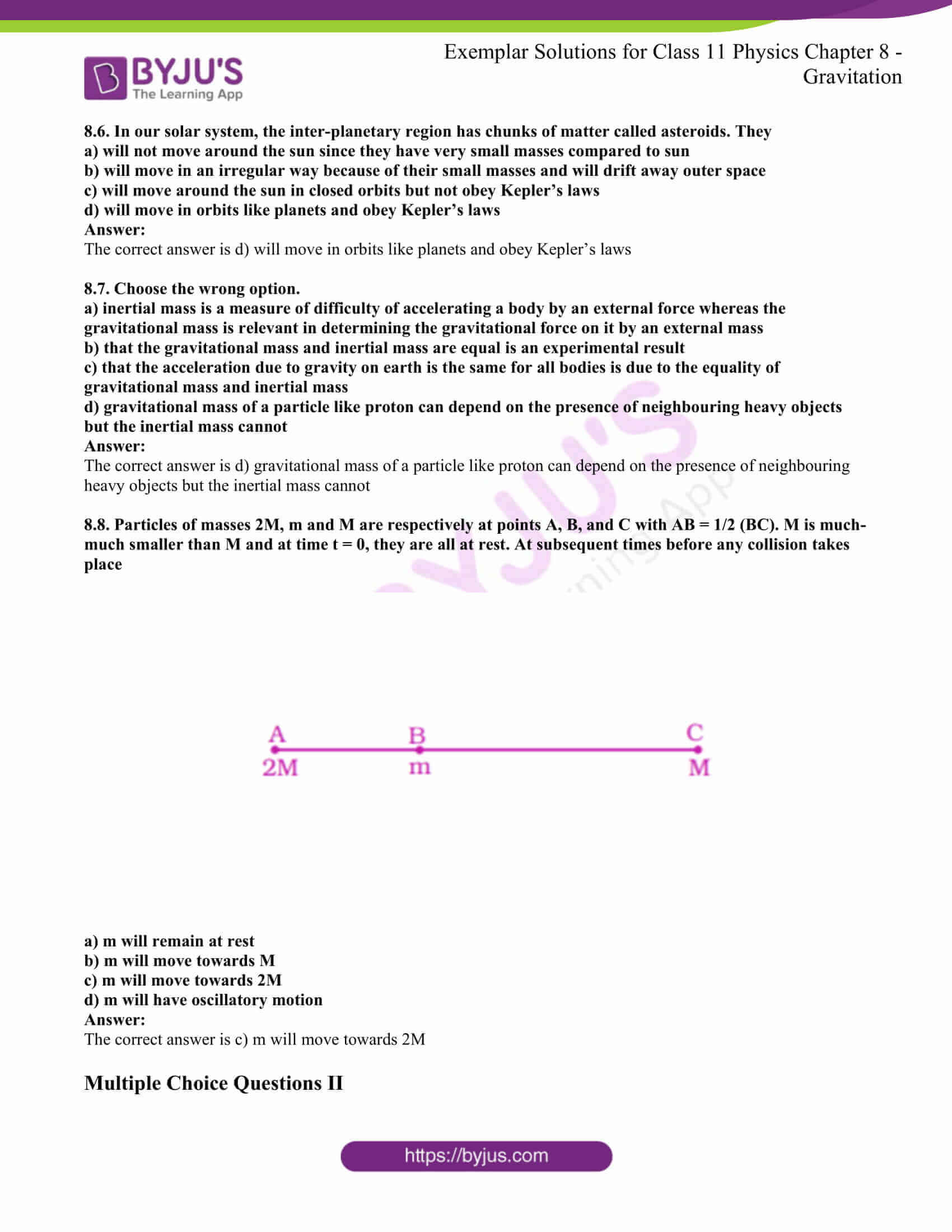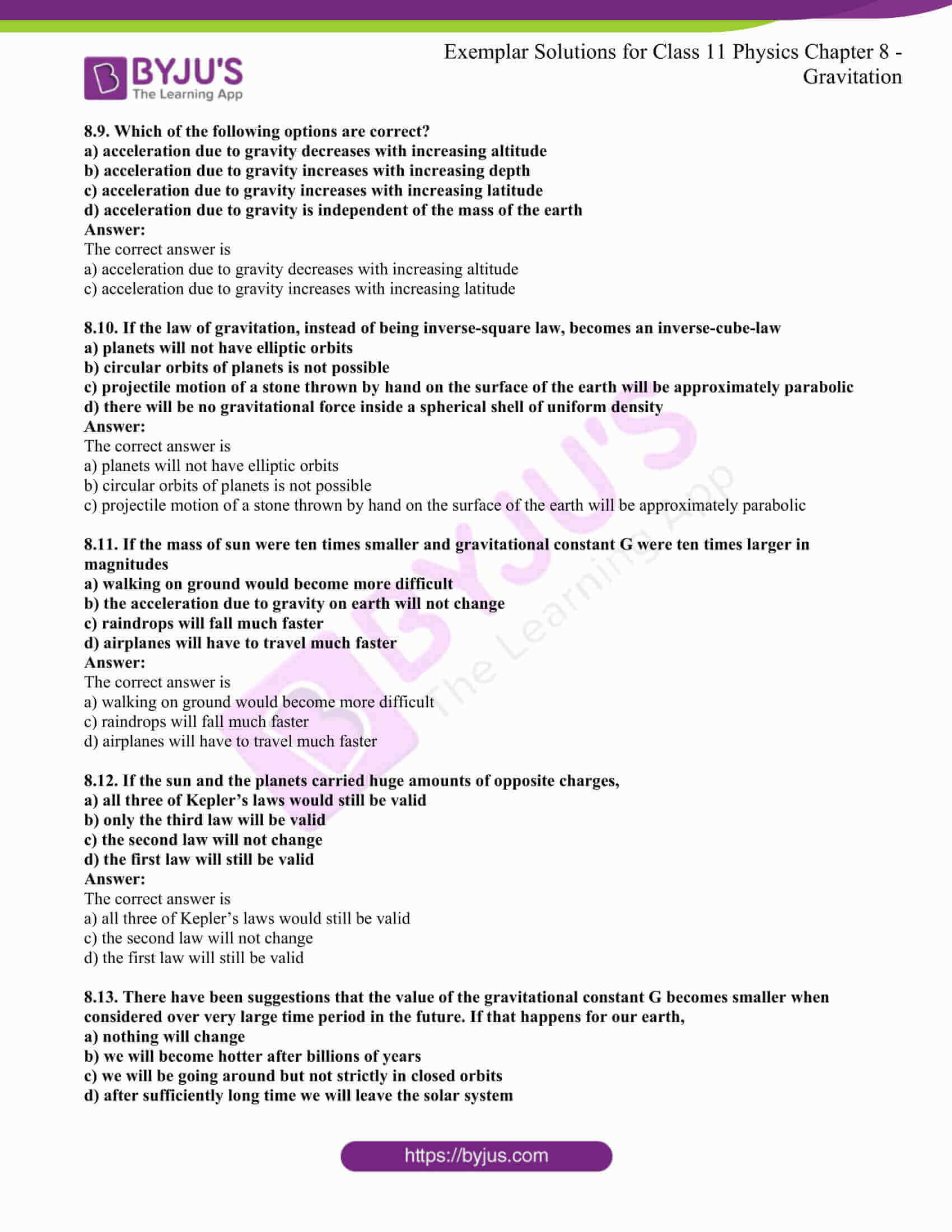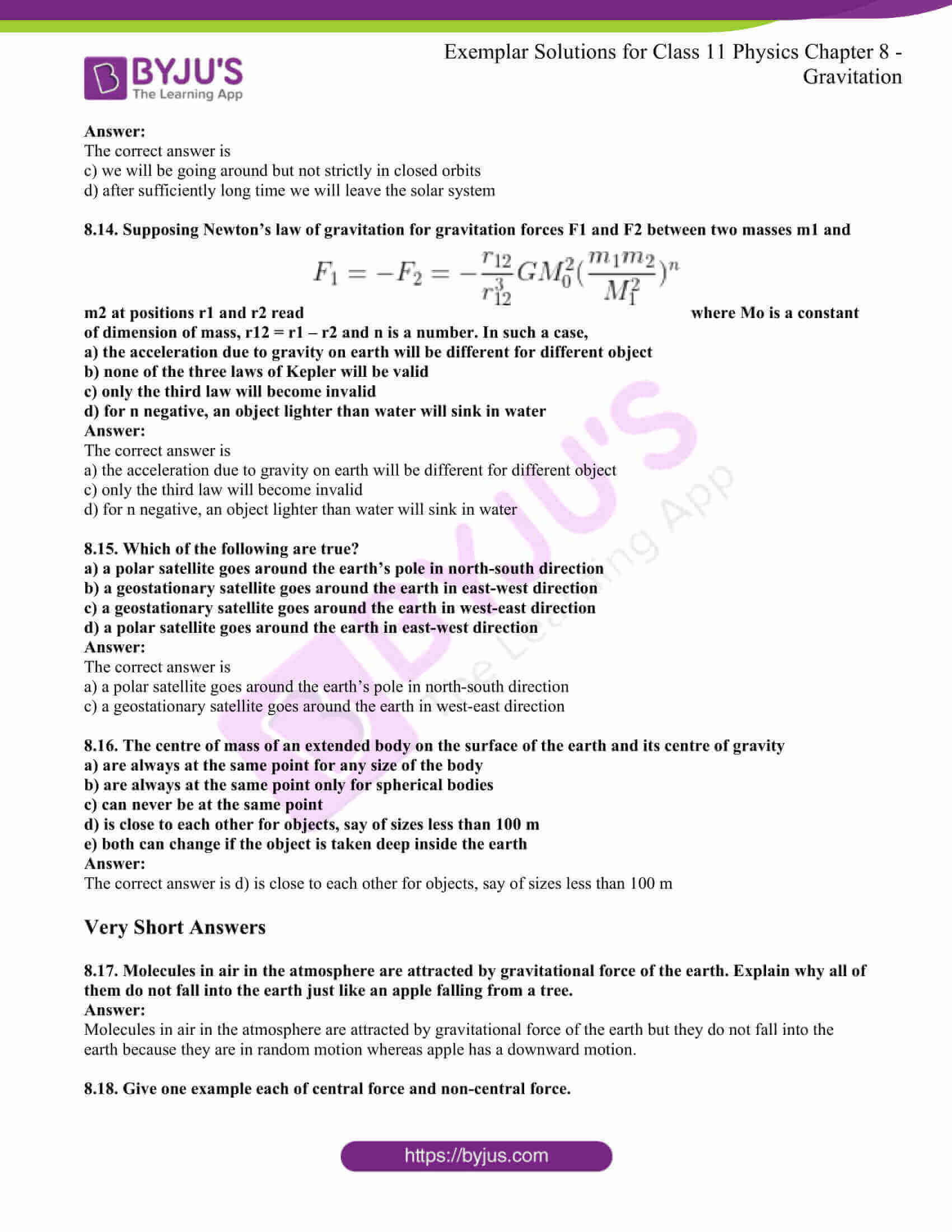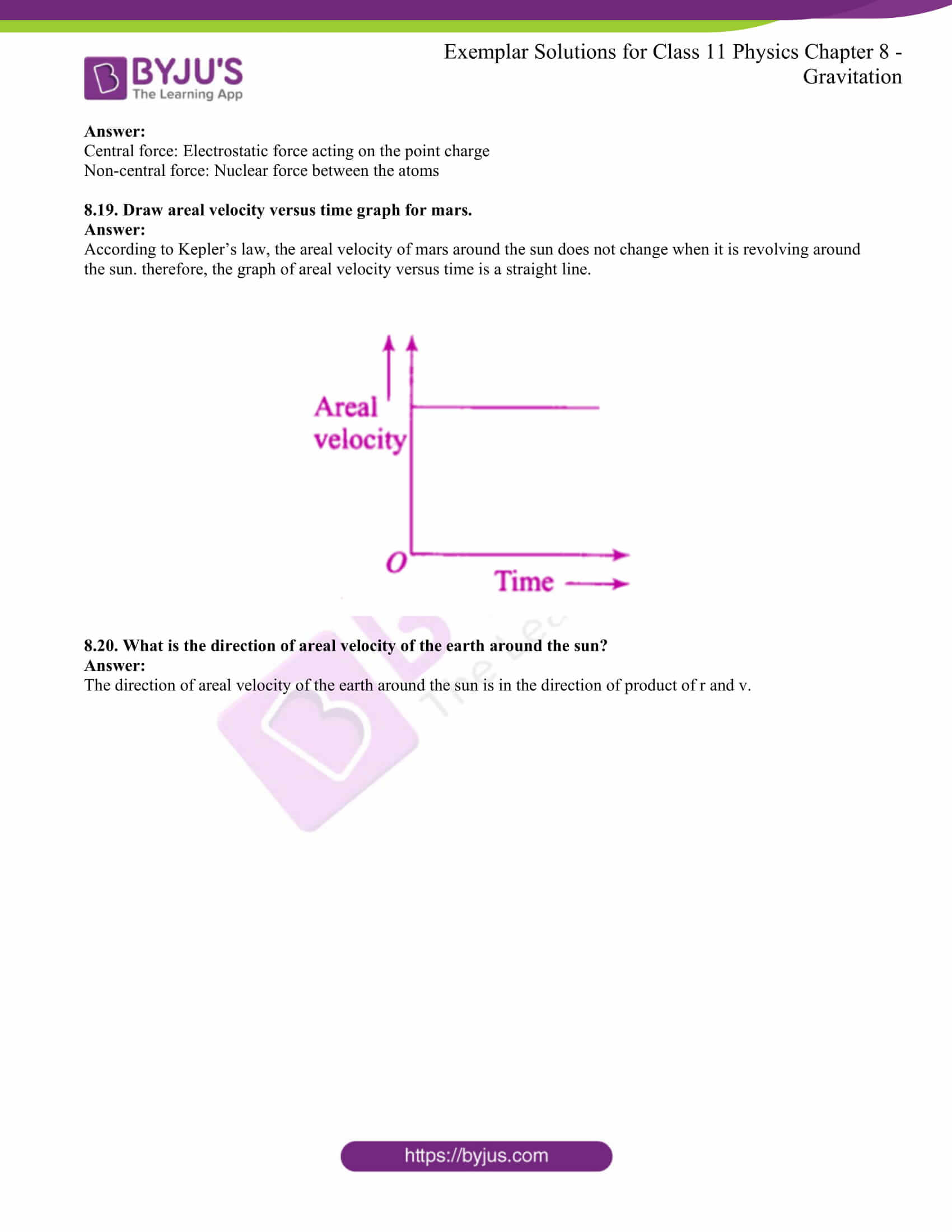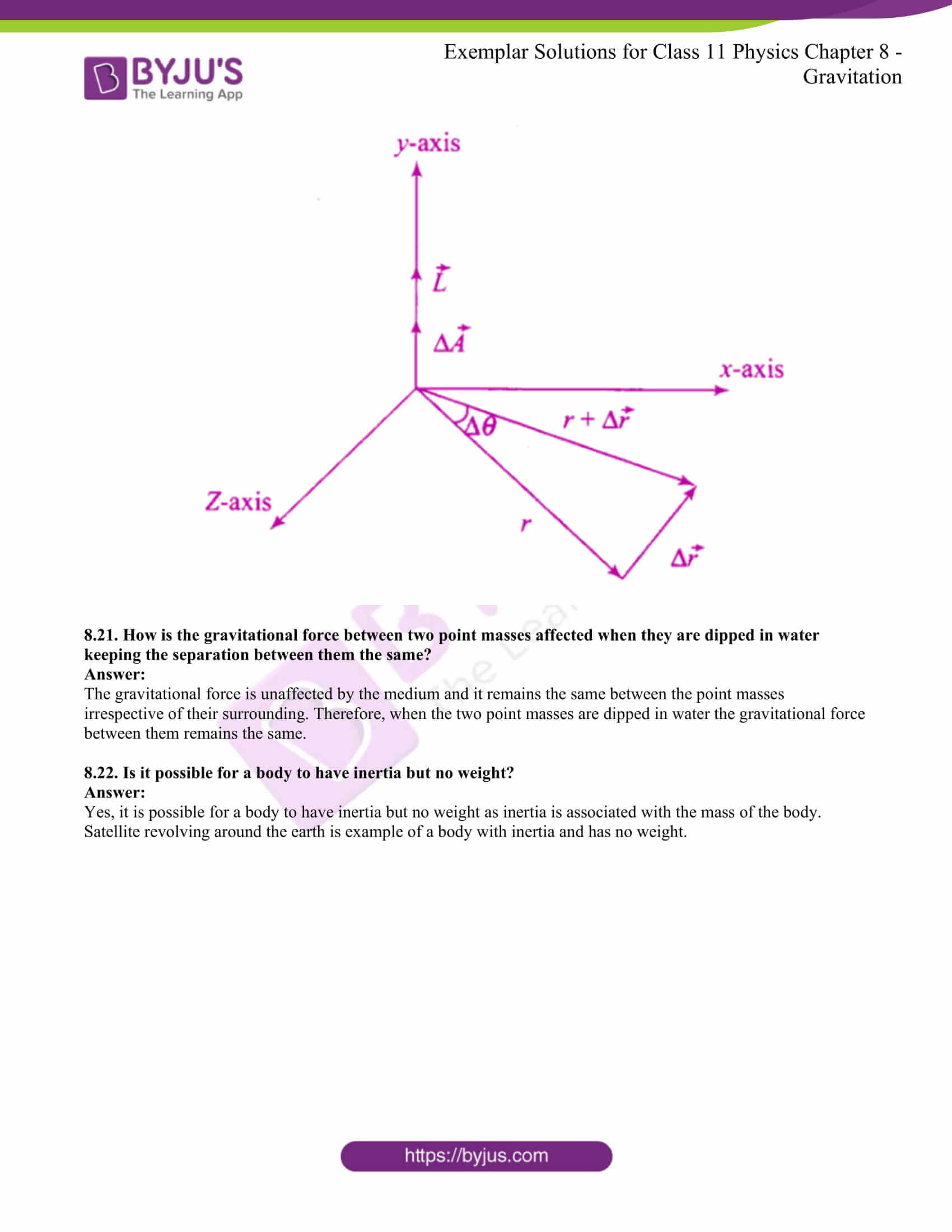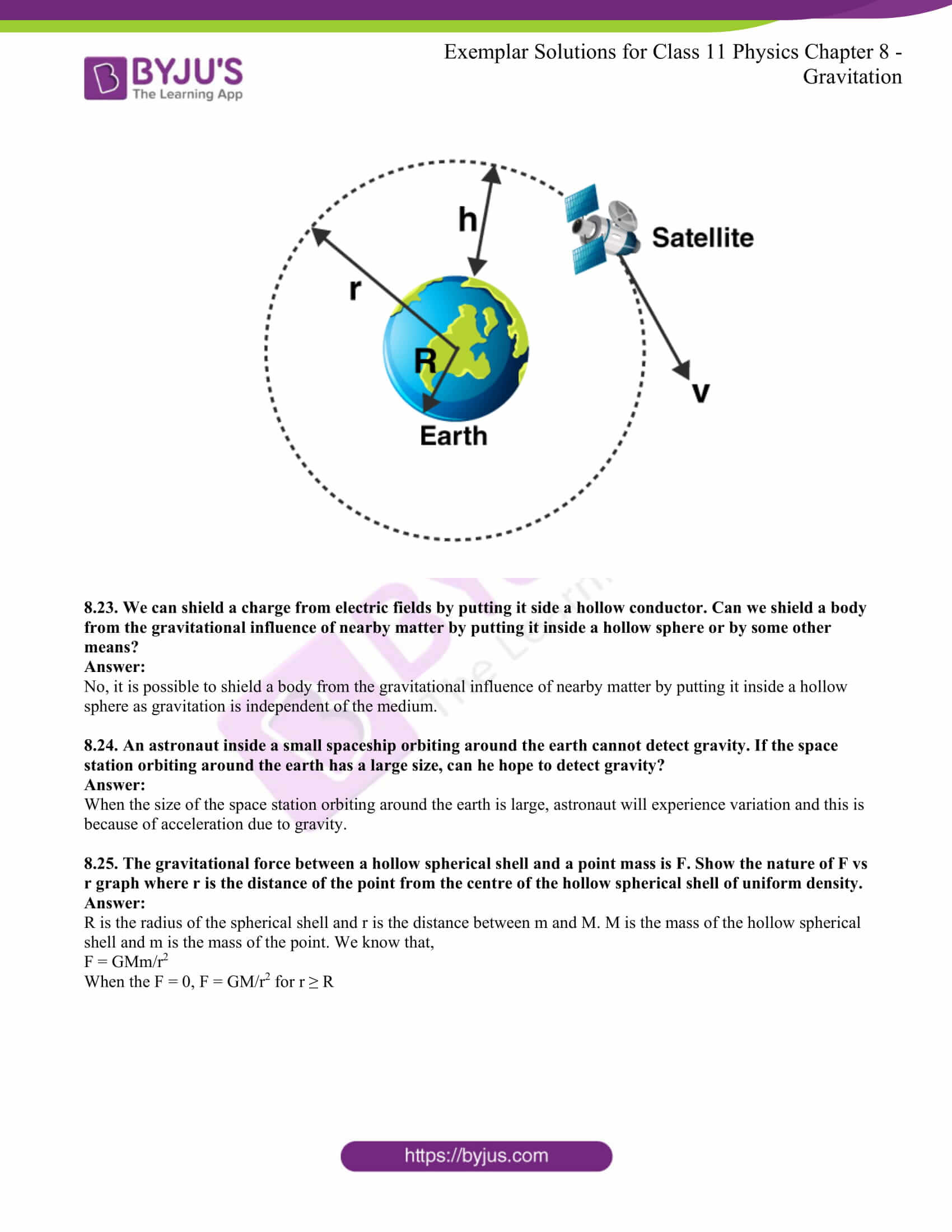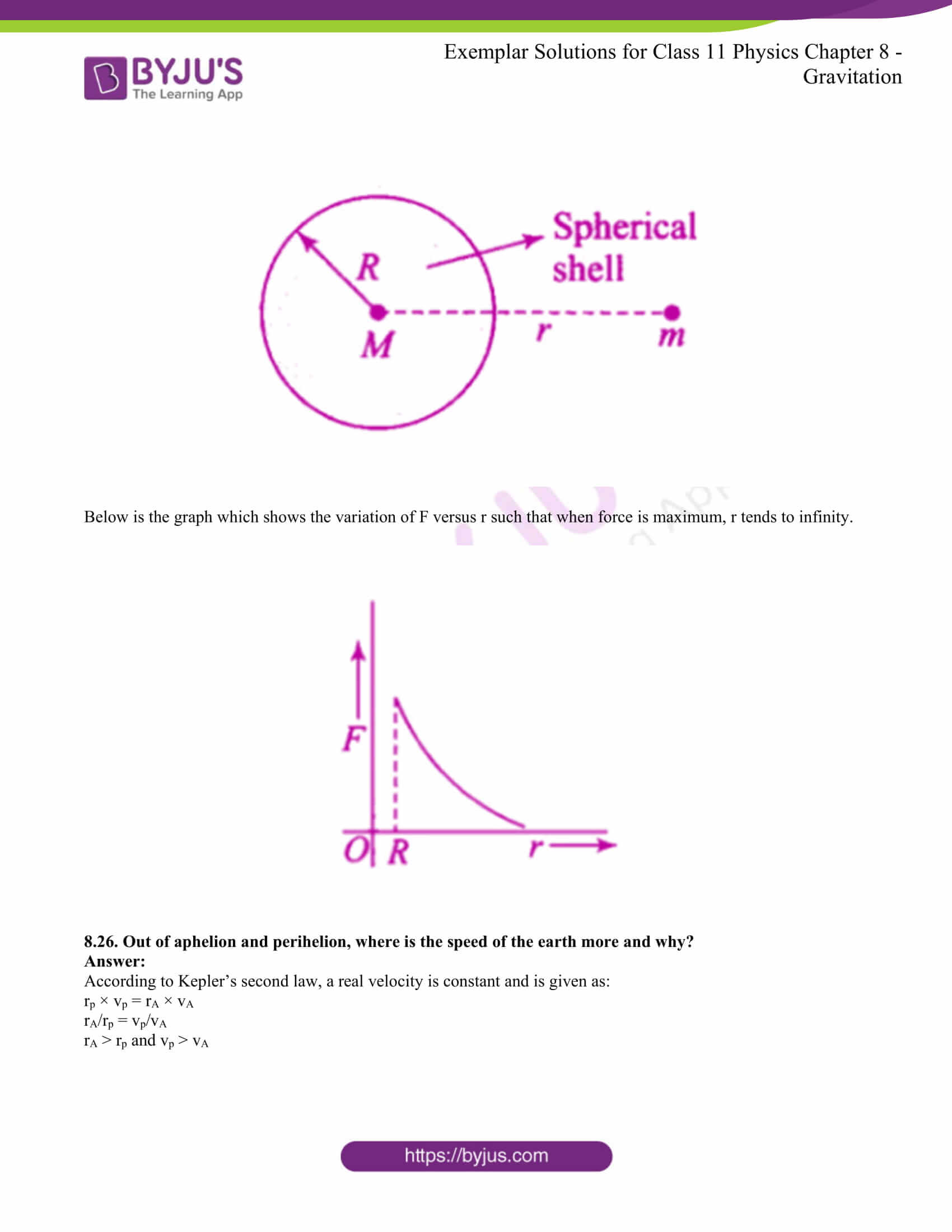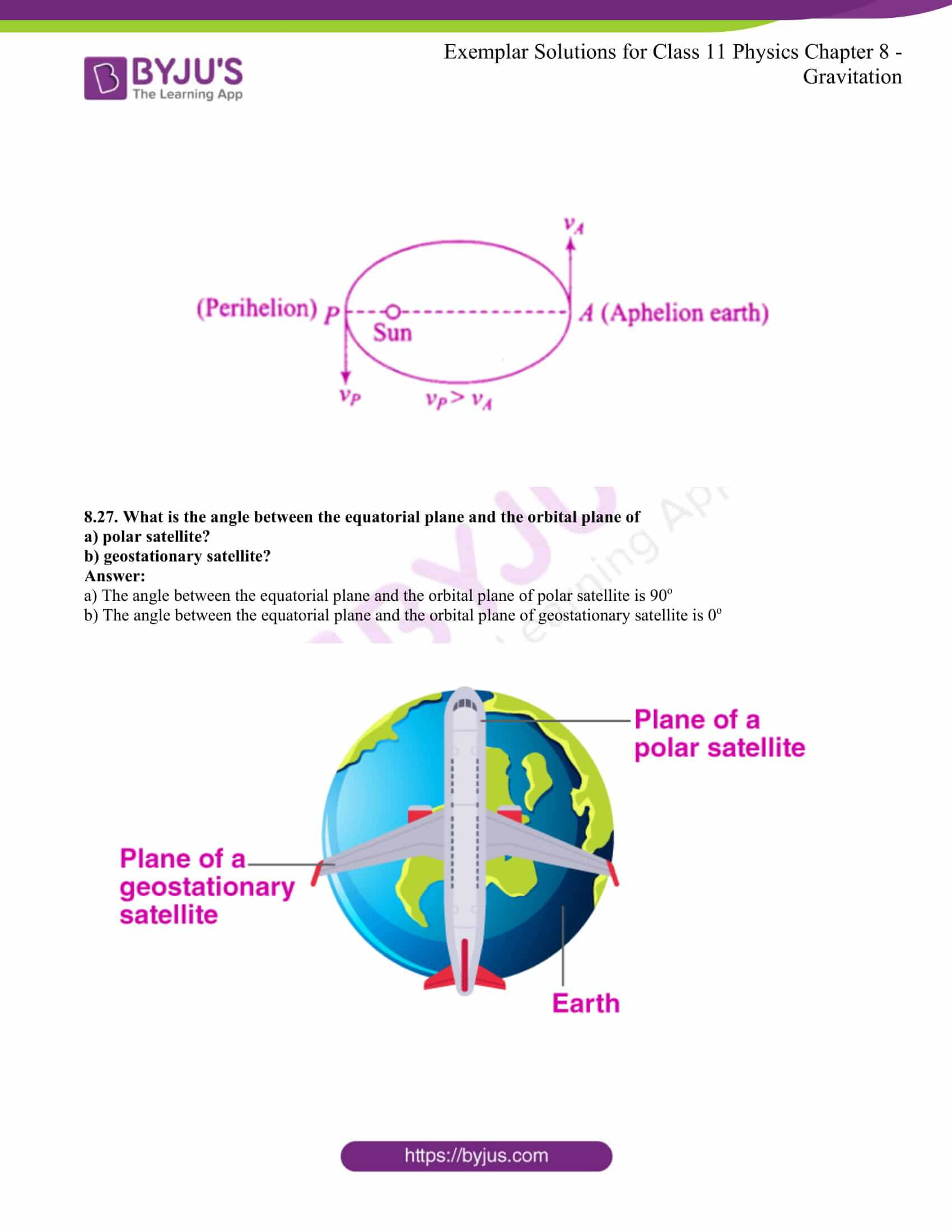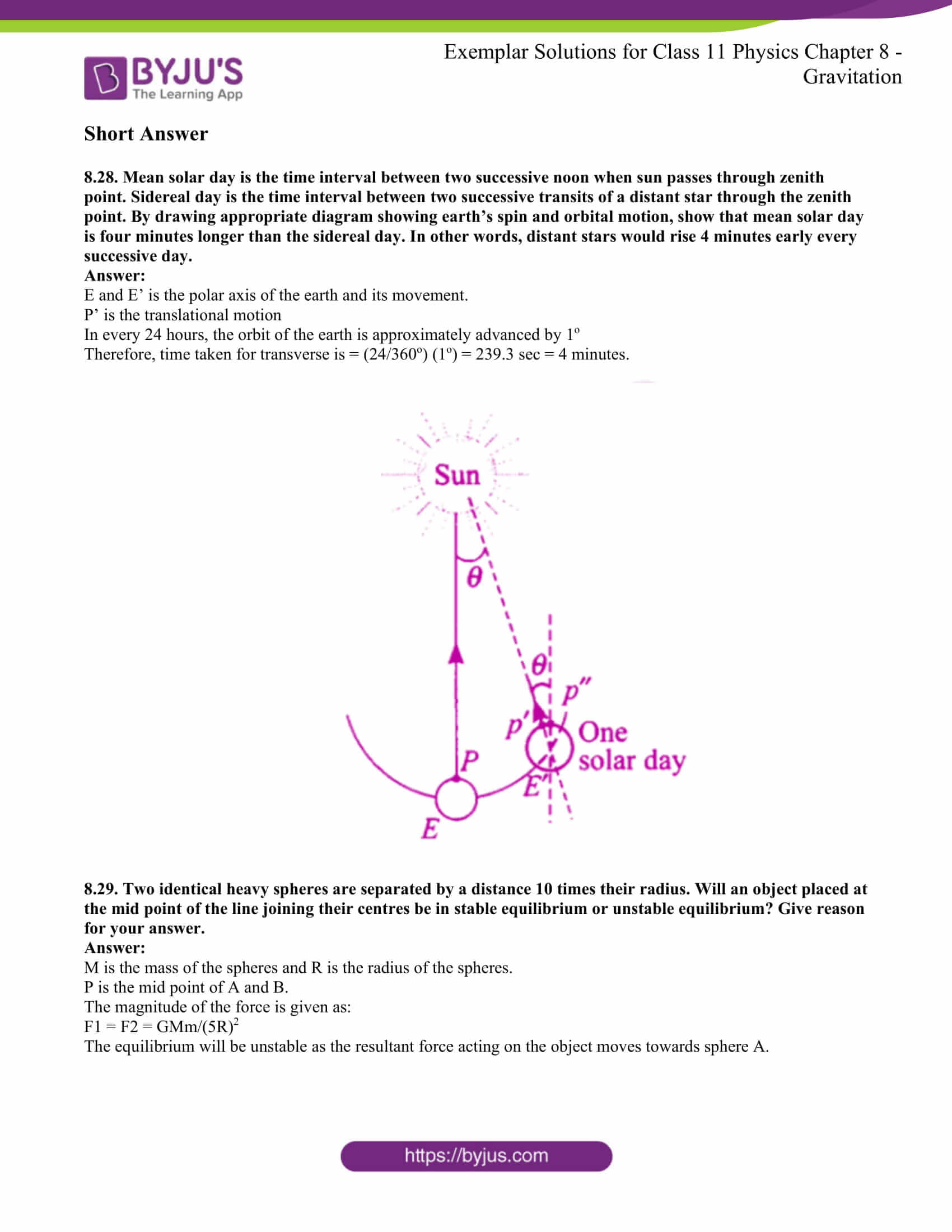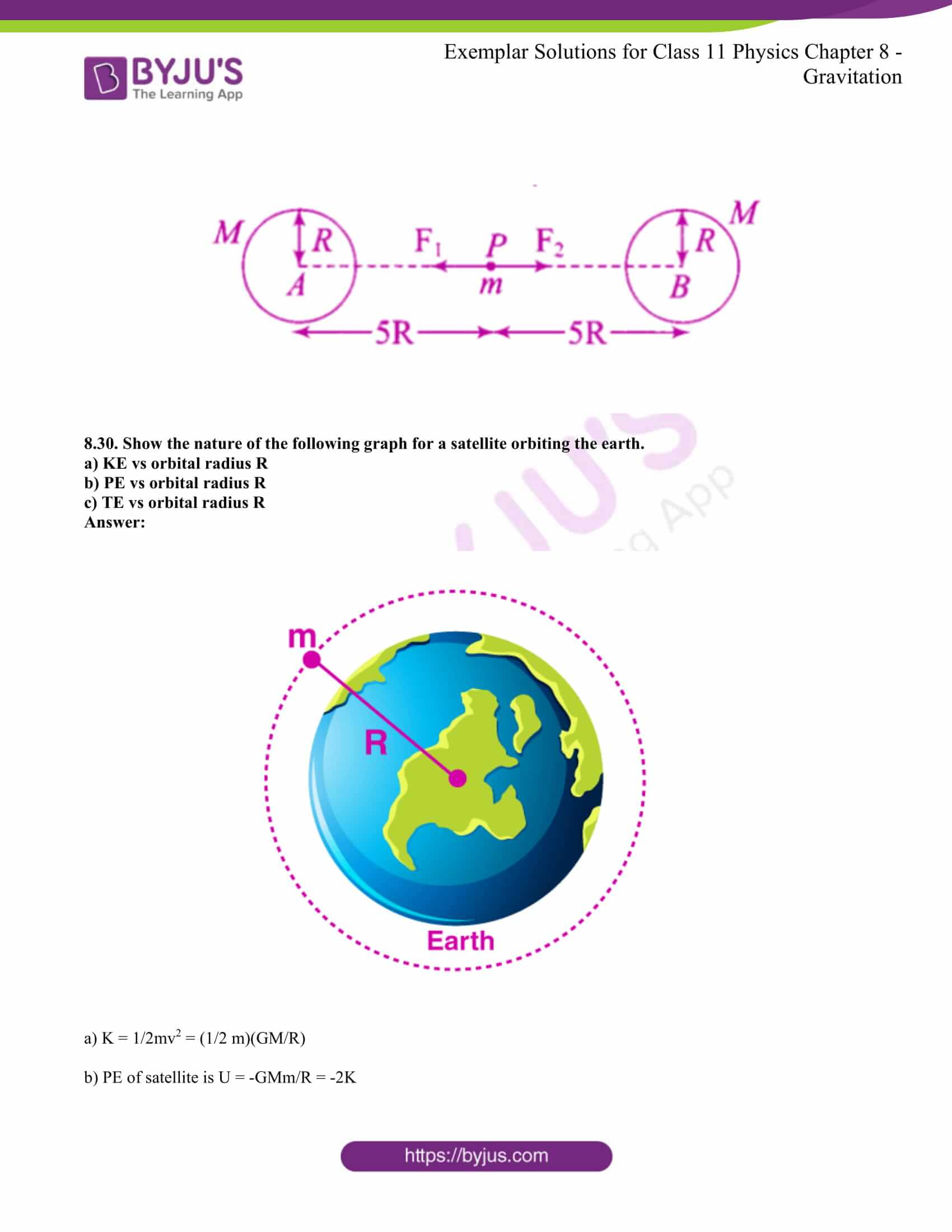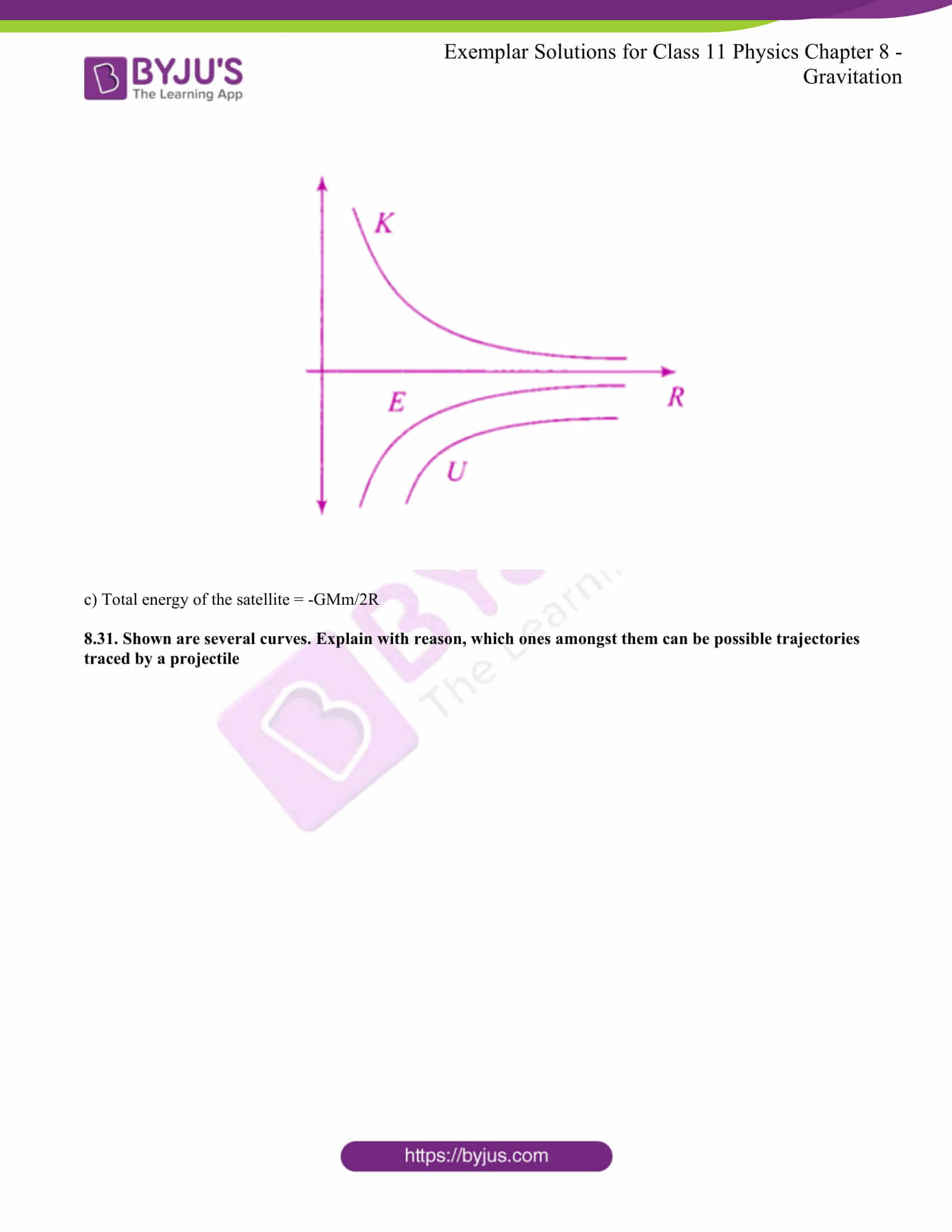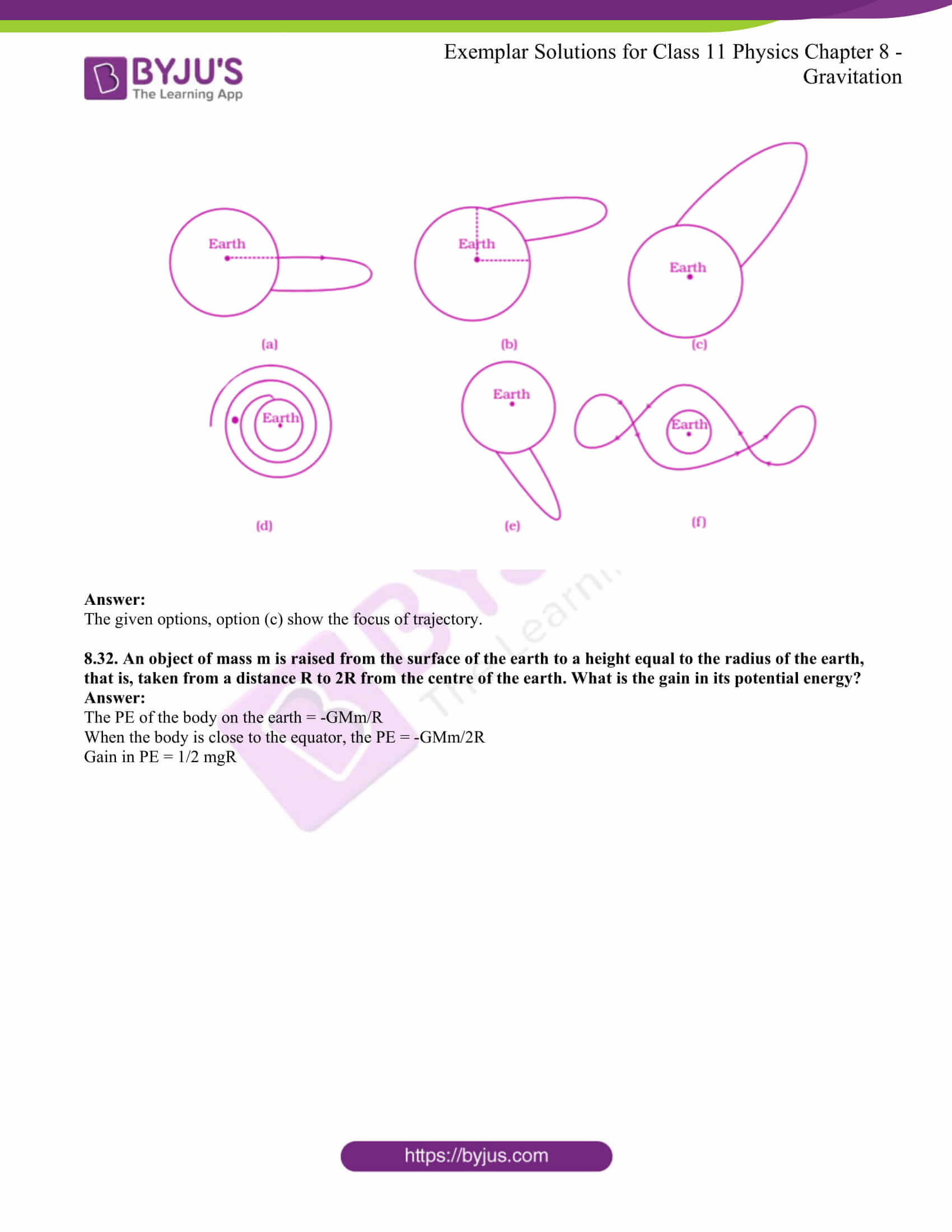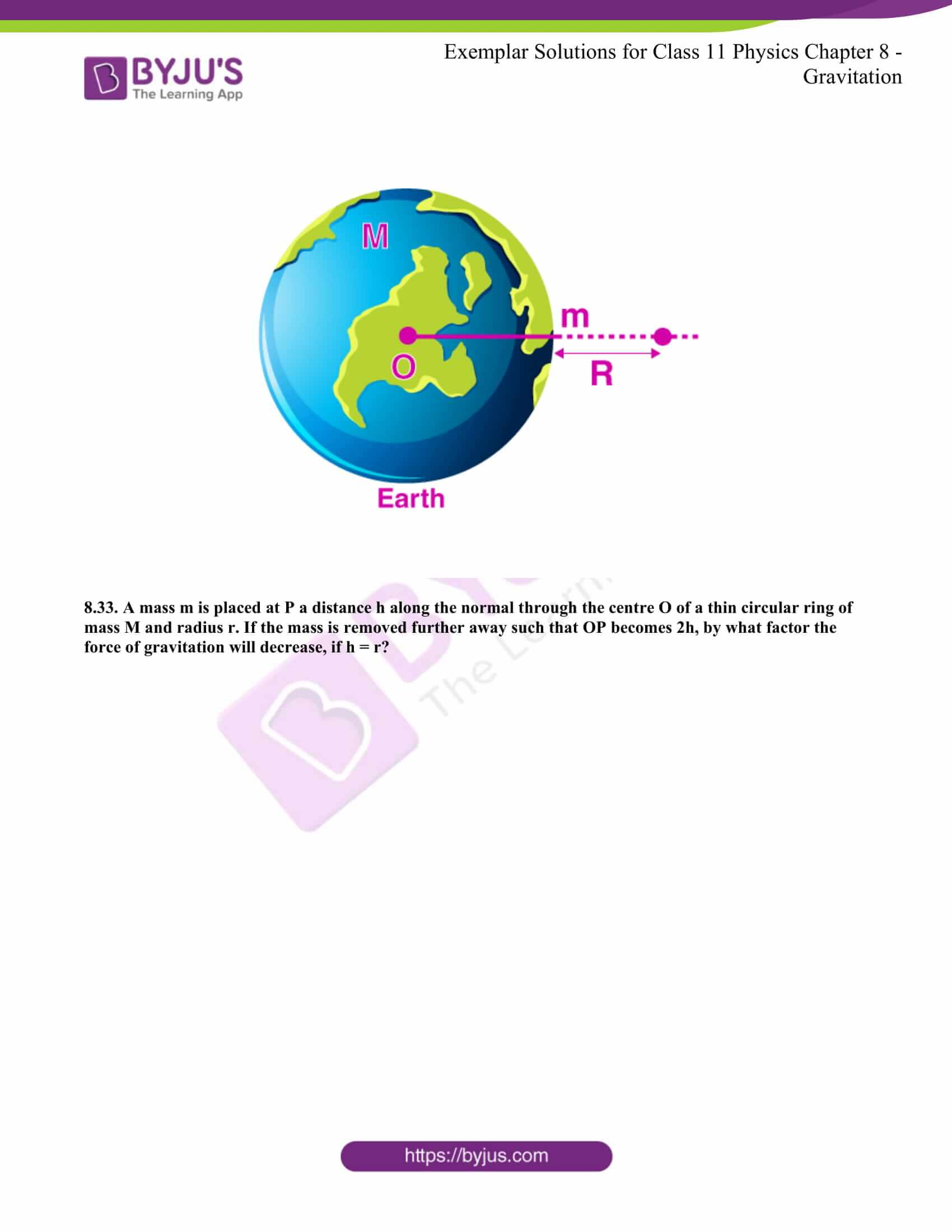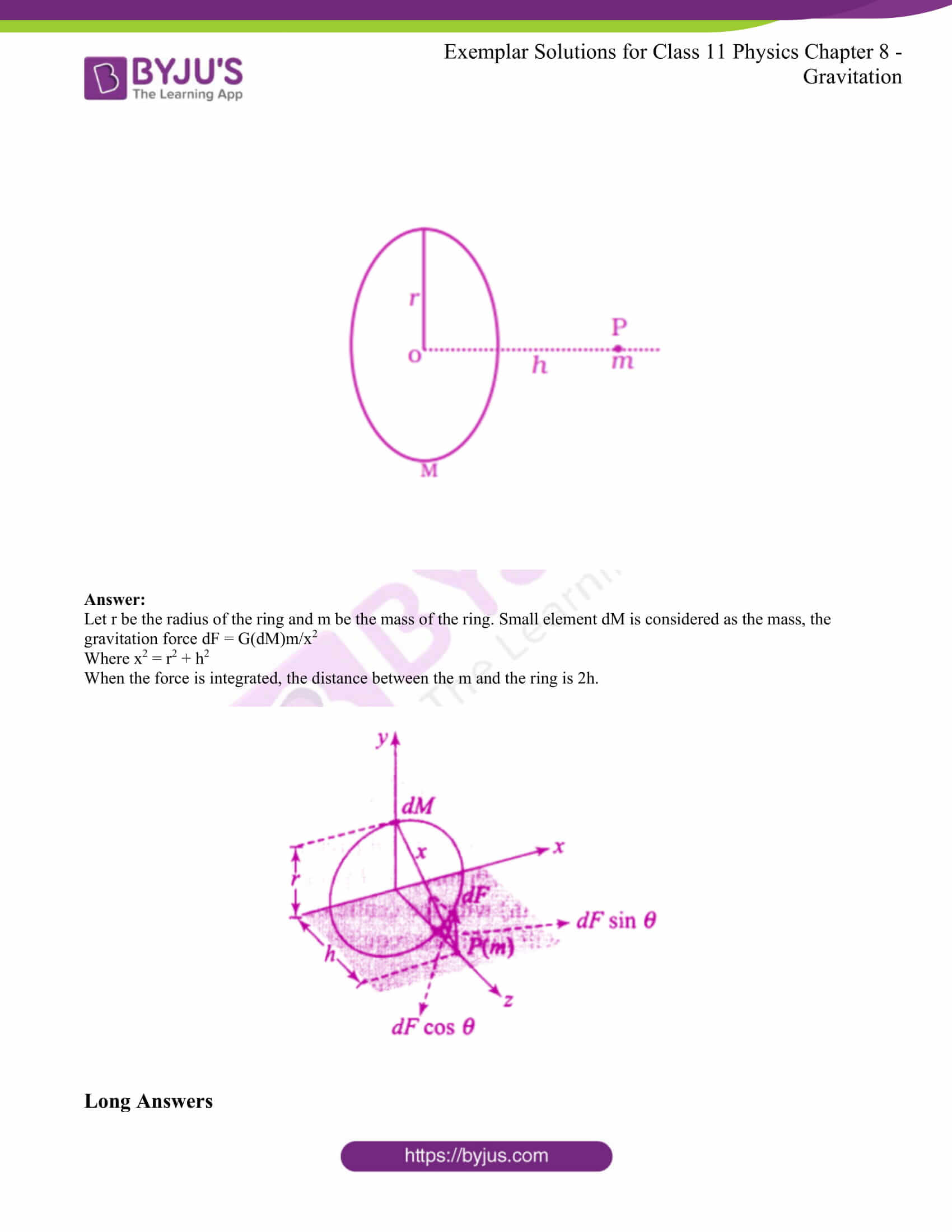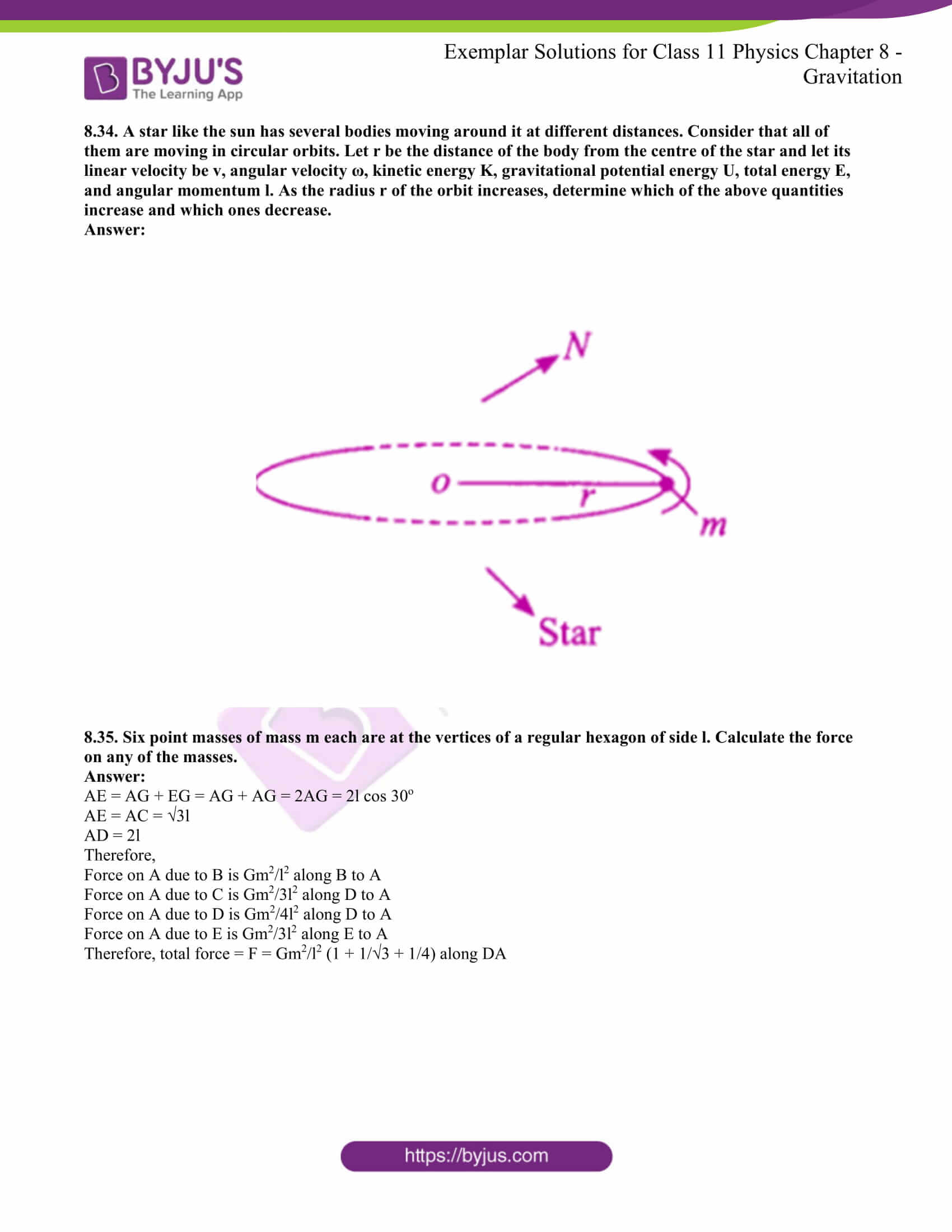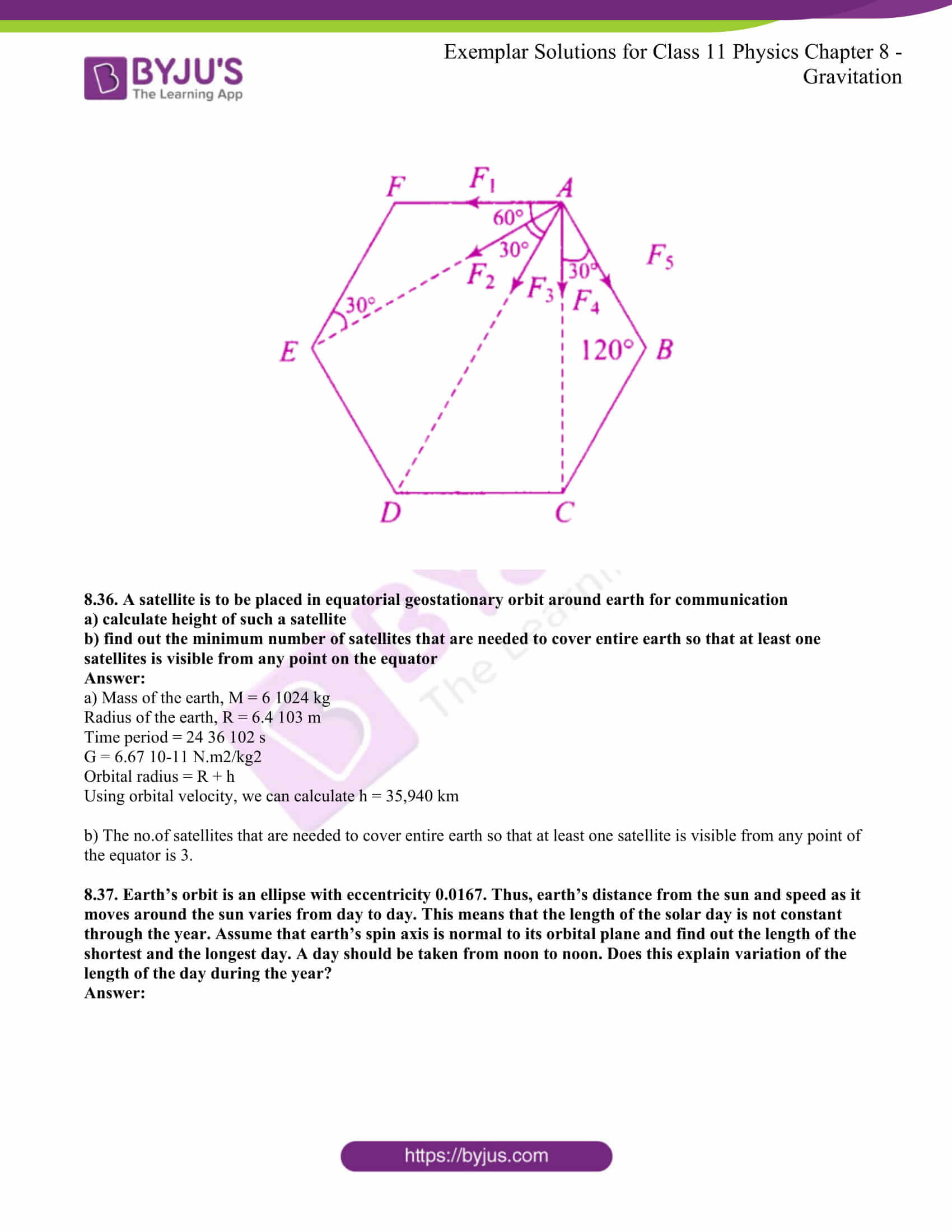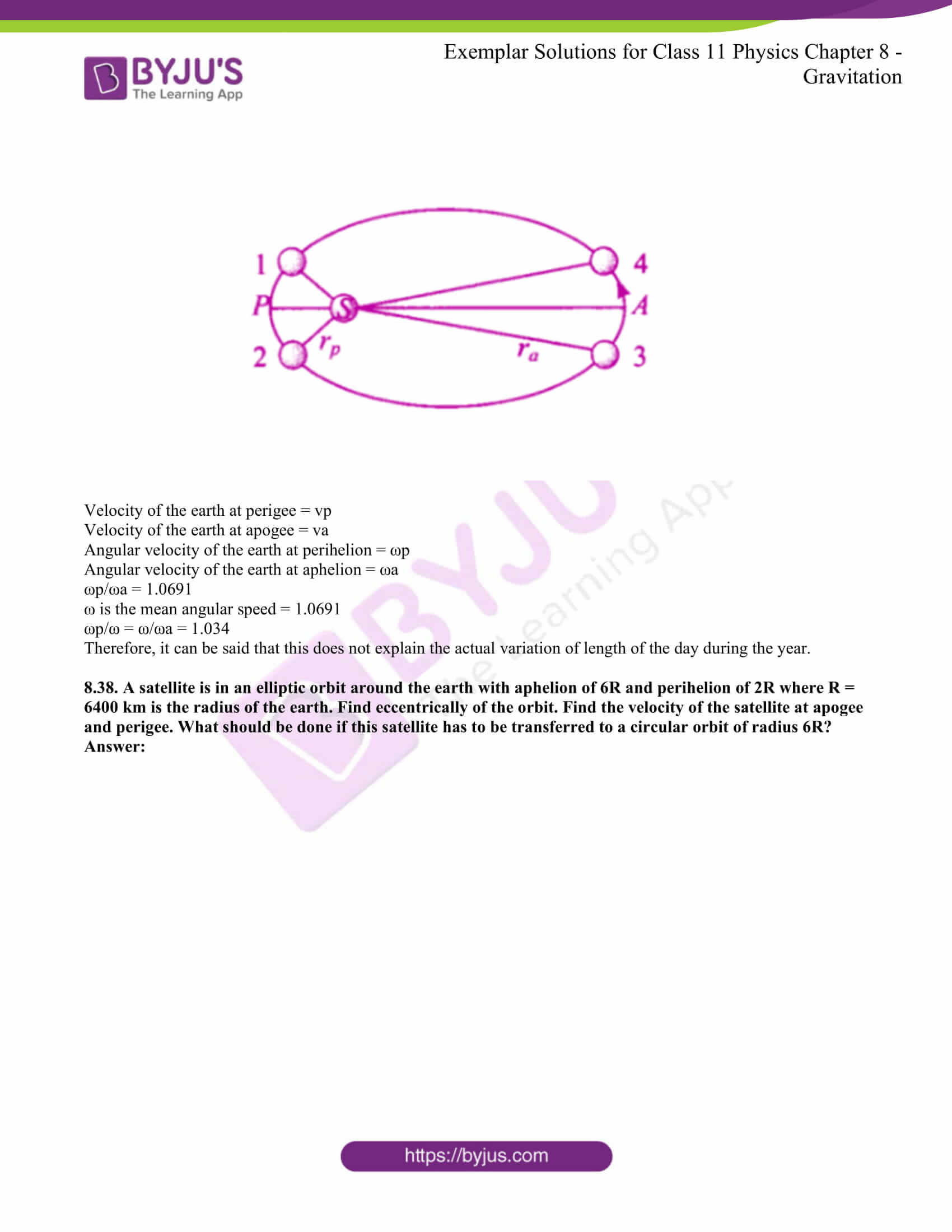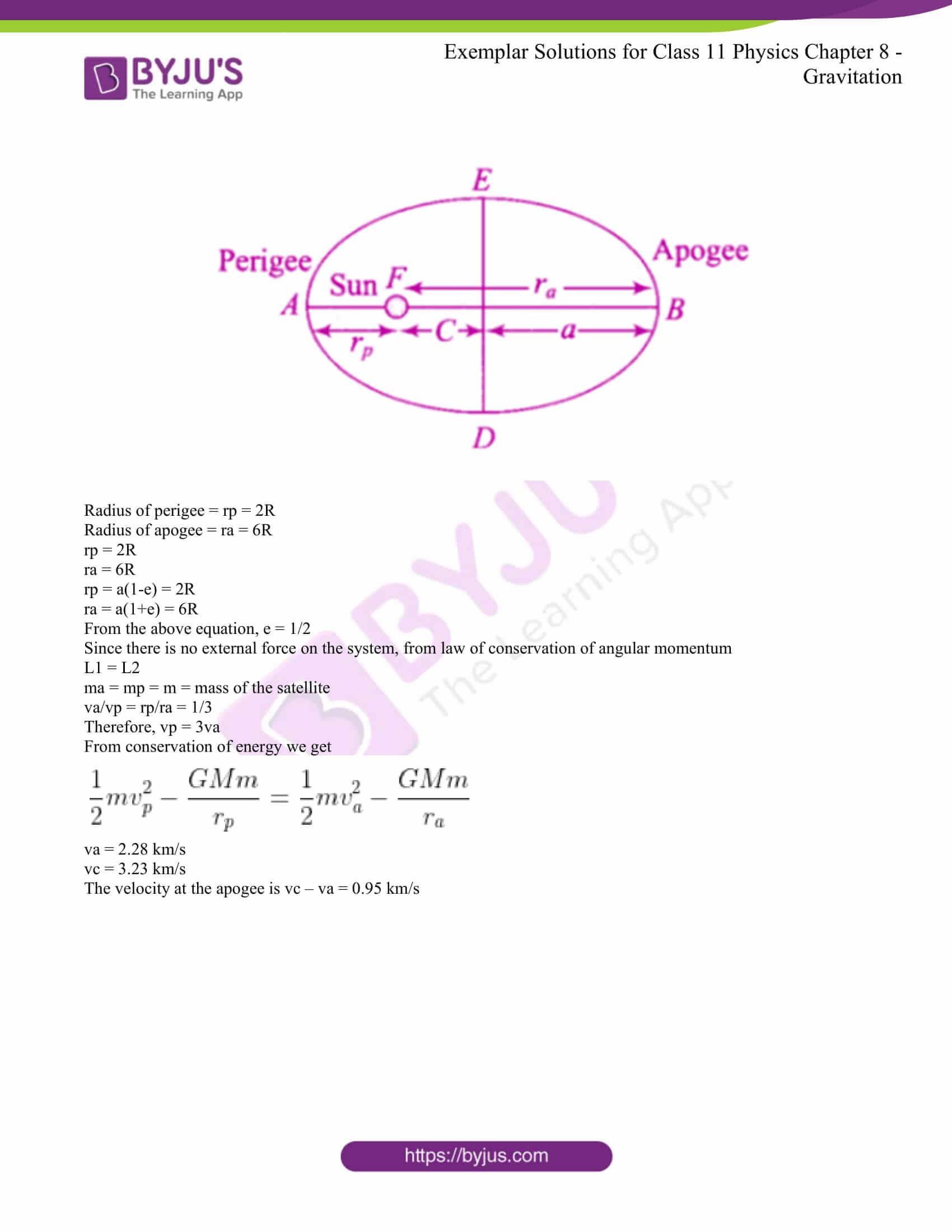### Access Answers to NCERT Exemplar class 11 Physics Chapter 8

#### Multiple Choice Questions I

8.1. The earth is an approximate sphere. If the interior contained matter which is not of the same density everywhere, then on the surface of the earth, the acceleration due to gravity

a) will be directed towards the centre but not the same everywhere

b) will have the same value everywhere but not directed towards the centre

c) will be same everywhere in magnitude directed towards the centre

d) cannot be zero at any point

The correct answer is d) cannot be zero at any point

8.2. As observed from earth, the sun appears to move in an approximately circular orbit. For the motion of another planet like mercury as observed from earth, this would

a) be similarly true

b) not be true because the force between earth and mercury is not inverse square law

c) not be true because the major gravitational force on mercury is due to sun

d) not be true because mercury is influenced by forces other than gravitational forces

The correct answer is c) not be true because the major gravitational force on mercury is due to sun

8.3. Different points in the earth are at slightly different distances from the sun and hence experience different forces due to gravitation. For a rigid body, we know that if various forces act at various points in it, the resultant motion is as if a net force acts on the cm causing translation and a net torque at the cm causing translation and a net torque at the cm causing rotation around an axis through the cm. For the earth-sun system

a) the torque is zero

b) the torque causes the earth to spin

c) the rigid body result is not applicable since the earth is not even approximately a rigid body

d) the torque causes the earth to move around the sun

The correct answer is a) the torque is zero

8.4. Satellites orbiting the earth have a finite life and sometimes debris of satellites fall to the earth. This is because

a) the solar cells and batteries in satellites run out

b) the laws of gravitation predict a trajectory spiralling inwards

c) of viscous forces causing the speed of the satellite and hence height to gradually decrease

d) of collisions with other satellites

The correct answer is c) of viscous forces causing the speed of the satellite and hence height to gradually decrease

8.5. Both earth and moon are subject to the gravitational force of the sun. as observed from the sun, the orbit of the moon

a) will be elliptical

b) will not be strictly elliptical because the total gravitational force on it is not central

c) is not elliptical but will necessarily be a closed curve

d) deviates considerably from being elliptical due to the influence of planets other than earth

The correct answer is b) will not be strictly elliptical because the total gravitational force on it is not central

8.6. In our solar system, the inter-planetary region has chunks of matter called asteroids. They

a) will not move around the sun since they have very small masses compared to the sun

b) will move in an irregular way because of their small masses and will drift away outer space

c) will move around the sun in closed orbits but not obey Kepler’s laws

d) will move in orbits like planets and obey Kepler’s laws

The correct answer is d) will move in orbits like planets and obey Kepler’s laws

8.7. Choose the wrong option.

a) inertial mass is a measure of the difficulty of accelerating a body by an external force whereas the gravitational mass is relevant in determining the gravitational force on it by an external mass

b) that the gravitational mass and inertial mass are equal is an experimental result

c) that the acceleration due to gravity on earth is the same for all bodies is due to the equality of gravitational mass and inertial mass

d) gravitational mass of a particle-like proton can depend on the presence of neighbouring heavy objects but the inertial mass cannot

The correct answer is d) gravitational mass of a particle-like proton can depend on the presence of neighbouring heavy objects but the inertial mass cannot

8.8. Particles of masses 2M, m and M are respectively at points A, B, and C with AB = 1/2 (BC). M is much-much smaller than M and at time t = 0, they are all at rest. At subsequent times before any collision takes place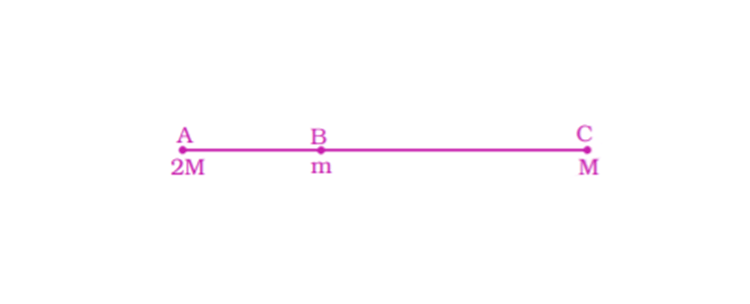a) m will remain at rest

b) m will move towards M

c) m will move towards 2M

d) m will have oscillatory motion

The correct answer is c) m will move towards 2M

### Multiple Choice Questions II

8.9. Which of the following options is correct?

a) acceleration due to gravity decreases with increasing altitude

b) acceleration due to gravity increases with increasing depth

c) acceleration due to gravity increases with increasing latitude

d) acceleration due to gravity is independent of the mass of the earth

a) acceleration due to gravity decreases with increasing altitude

c) acceleration due to gravity increases with increasing latitude

8.10. If the law of gravitation, instead of being inverse-square law, becomes an inverse-cube-law

a) planets will not have elliptic orbits

b) circular orbits of planets is not possible

c) projectile motion of a stone thrown by hand on the surface of the earth will be approximately parabolic

d) there will be no gravitational force inside a spherical shell of uniform density

a) planets will not have elliptic orbits

b) circular orbits of planets is not possible

c) projectile motion of a stone thrown by hand on the surface of the earth will be approximately parabolic

8.11. If the mass of sun were ten times smaller and gravitational constant G were ten times larger in magnitudes

a) walking on ground would become more difficult

b) the acceleration due to gravity on earth will not change

c) raindrops will fall much faster

d) aeroplanes will have to travel much faster

a) walking on ground would become more difficult

c) raindrops will fall much faster

d) aeroplanes will have to travel much faster

8.12. If the sun and the planets carried huge amounts of opposite charges,

a) all three of Kepler’s laws would still be valid

b) only the third law will be valid

c) the second law will not change

d) the first law will still be valid

a) all three of Kepler’s laws would still be valid

c) the second law will not change

d) the first law will still be valid

8.13. There have been suggestions that the value of the gravitational constant G becomes smaller when considered over a very large time period in the future. If that happens for our earth,

a) nothing will change

b) we will become hotter after billions of years

c) we will be going around but not strictly in closed orbits

d) after a sufficiently long time we will leave the solar system

c) we will be going around but not strictly in closed orbits

d) after a sufficiently long time we will leave the solar system

8.14. Supposing Newton’s law of gravitation for gravitation forces F1 and F2 between two masses m1 and m2 at positions r1 and r2 read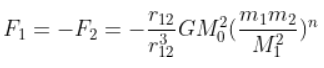where Mo is a constant of the dimension of mass, r12 = r1 – r2 and n is a number. In such a case,

a) the acceleration due to gravity on earth will be different for different object

b) none of the three laws of Kepler will be valid

c) only the third law will become invalid

d) for n negative, an object lighter than water will sink in water

a) the acceleration due to gravity on earth will be different for different object

c) only the third law will become invalid

d) for n negative, an object lighter than water will sink in water

8.15. Which of the following are true?

a) a polar satellite goes around the earth’s pole in a north-south direction

b) a geostationary satellite goes around the earth in an east-west direction

c) a geostationary satellite goes around the earth in a west-east direction

d) a polar satellite goes around the earth in an east-west direction

a) a polar satellite goes around the earth’s pole in a north-south direction

c) a geostationary satellite goes around the earth in a west-east direction

8.16. The centre of mass of an extended body on the surface of the earth and its centre of gravity

a) are always at the same point for any size of the body

b) are always at the same point only for spherical bodies

c) can never be at the same point

d) is close to each other for objects, say of sizes less than 100 m

e) both can change if the object is taken deep inside the earth

The correct answer is d) is close to each other for objects, say of sizes less than 100 m

8.17. Molecules in the air in the atmosphere are attracted by the gravitational force of the earth. Explain why all of them do not fall into the earth just like an apple falling from a tree.

Molecules in the air in the atmosphere are attracted by the gravitational force of the earth but they do not fall into the earth because they are in random motion whereas apple has a downward motion.

8.18. Give one example each of central force and non-central force.

Central force: Electrostatic force acting on the point charge

Non-central force: Nuclear force between the atoms

8.19. Draw areal velocity versus time graph for mars.

According to Kepler’s law, the areal velocity of mars around the sun does not change when it is revolving around the sun. therefore, the graph of areal velocity versus time is a straight line.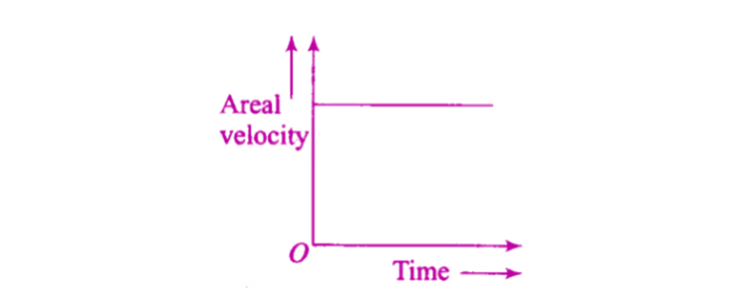8.20. What is the direction of areal velocity of the earth around the sun?

The direction of the areal velocity of the earth around the sun is in the direction of the product of r and v.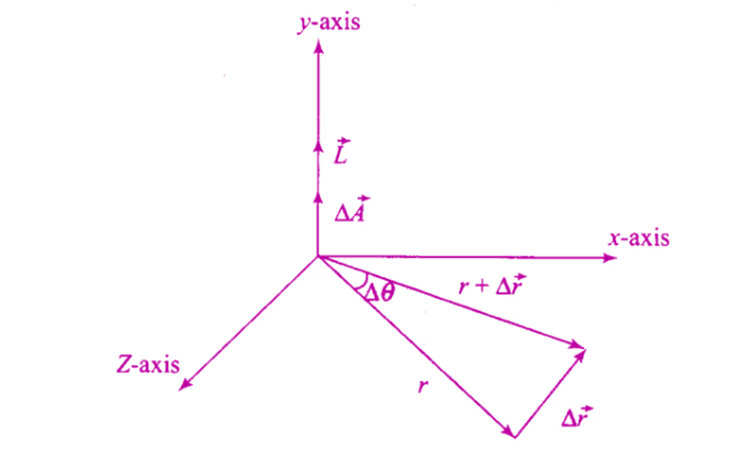8.21. How is the gravitational force between two point masses affected when they are dipped in the water keeping the separation between them the same?

The gravitational force is unaffected by the medium, and it remains the same between the point masses irrespective of their surrounding. Therefore, when the two-point masses are dipped in water, the gravitational force between them remains the same.

8.22. Is it possible for a body to have inertia but no weight?

Yes, it is possible for a body to have inertia but no weight as inertia is associated with the mass of the body. Satellite revolving around the earth is an example of a body with inertia and has no weight.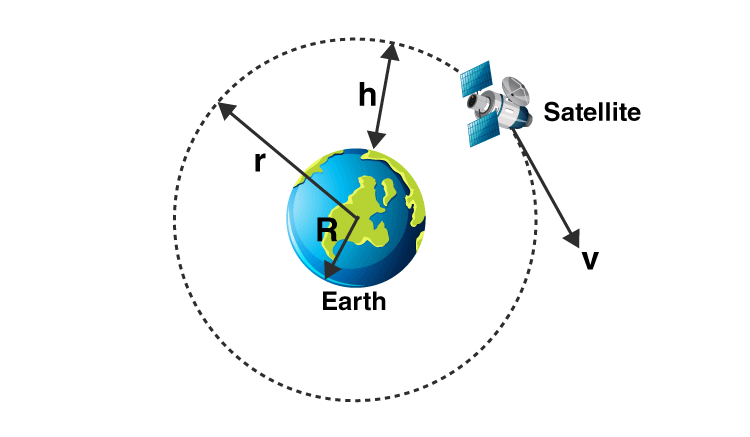8.23. We can shield a charge from electric fields by putting it side a hollow conductor. Can we shield a body from the gravitational influence of nearby matter by putting it inside a hollow sphere or by some other means?

No, it is possible to shield a body from the gravitational influence of nearby matter by putting it inside a hollow sphere as gravitation is independent of the medium.

8.24. An astronaut inside a small spaceship orbiting around the earth cannot detect gravity. If the space station orbiting around the earth has a large size, can he hope to detect gravity?

When the size of the space station orbiting around the earth is large, the astronaut will experience variation and this is because of acceleration due to gravity.

8.25. The gravitational force between a hollow spherical shell and a point mass is F. Show the nature of F vs r graph where r is the distance of the point from the centre of the hollow spherical shell of uniform density.

R is the radius of the spherical shell and r is the distance between m and M. M is the mass of the hollow spherical shell and m is the mass of the point. We know that,

F = GMm/r2

When the F = 0, F = GM/r2 for r ≥ R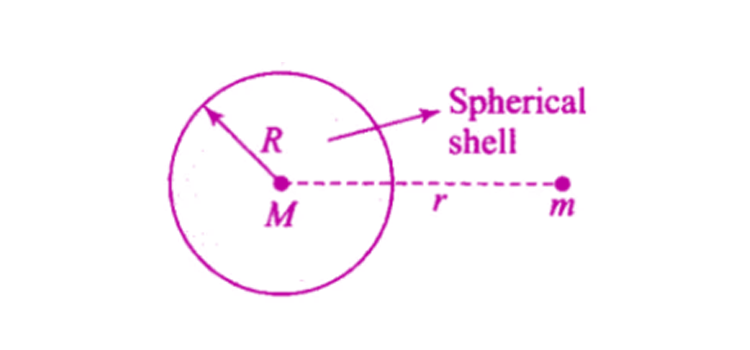Below is the graph which shows the variation of F versus r such that when force is maximum, r tends to infinity.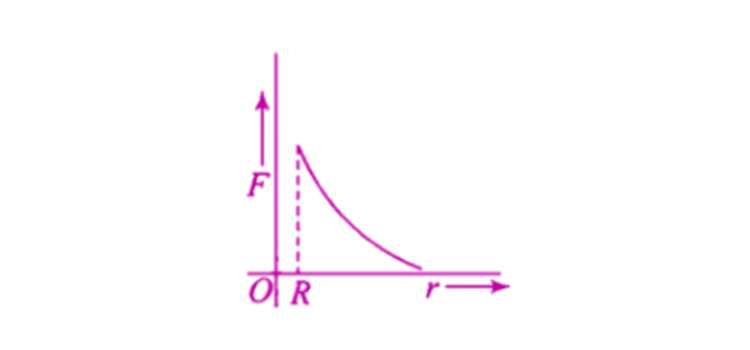8.26. Out of aphelion and perihelion, where is the speed of the earth more and why?

According to Kepler’s second law, a real velocity is constant and is given as:

rp × vp = rA × vA

rA/rp = vp/vA

rA > rp and vp > vA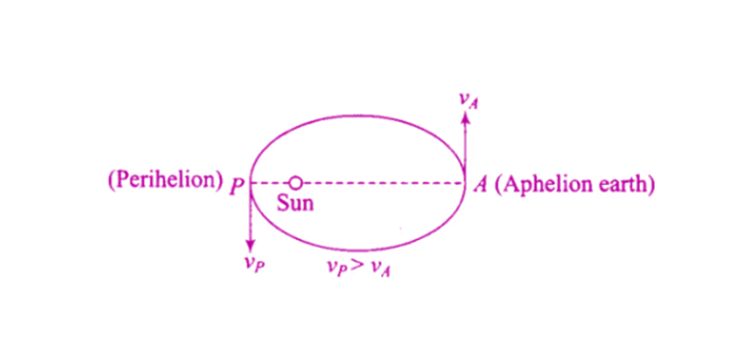8.27. What is the angle between the equatorial plane and the orbital plane of

a) polar satellite?

b) geostationary satellite?

a) The angle between the equatorial plane and the orbital plane of polar satellite is 90o

b) The angle between the equatorial plane and the orbital plane of a geostationary satellite is 0o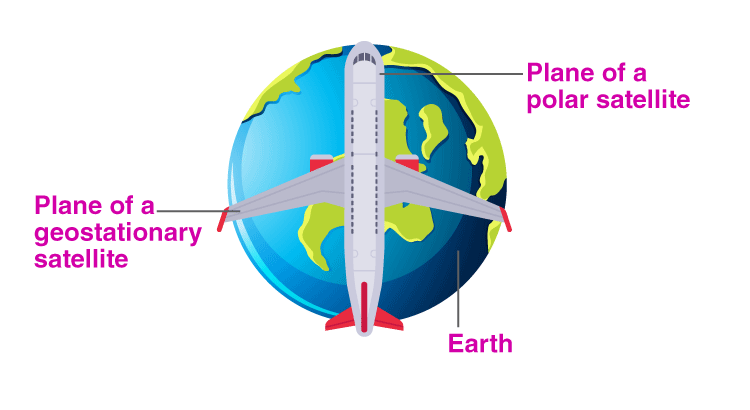8.28. Mean solar day is the time interval between two successive noon when the sun passes through zenith point. The sidereal day is the time interval between two successive transits of a distant star through the zenith point. By drawing the appropriate diagram showing earth’s spin and orbital motion, show that mean solar day is four minutes longer than the sidereal day. In other words, distant stars would rise 4 minutes early every successive day.

E and E’ is the polar axis of the earth and its movement.

P’ is the translational motion

In every 24 hours, the orbit of the earth is approximately advanced by 1o

Therefore, time taken for transverse is = (24/360o) (1o) = 239.3 sec = 4 minutes.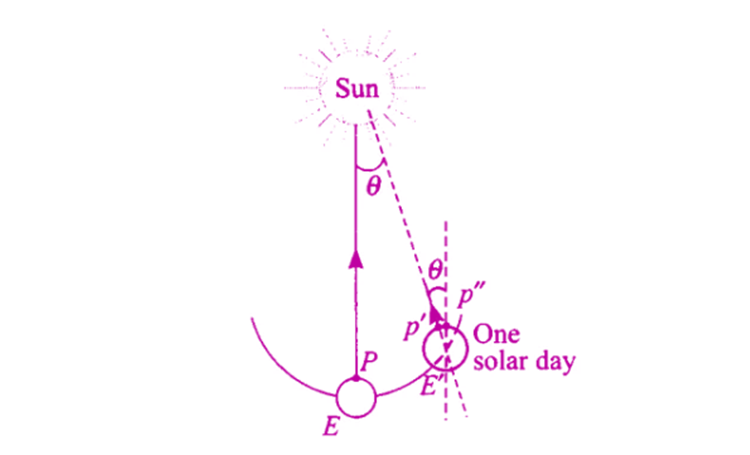8.29. Two identical heavy spheres are separated by a distance 10 times their radius. Will an object placed at the midpoint of the line joining their centres be in stable equilibrium or unstable equilibrium? Give a reason for your answer.

M is the mass of the spheres and R is the radius of the spheres.

P is the midpoint of A and B.

The magnitude of the force is given as:

F1 = F2 = GMm/(5R)2

The equilibrium will be unstable as the resultant force acting on the object moves towards sphere A.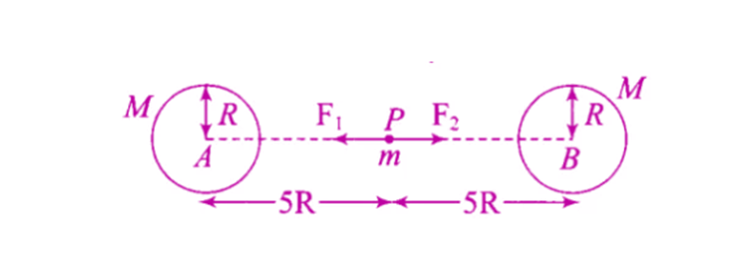8.30. Show the nature of the following graph for a satellite orbiting the earth.

a) KE vs orbital radius R

b) PE vs orbital radius R

c) TE vs orbital radius R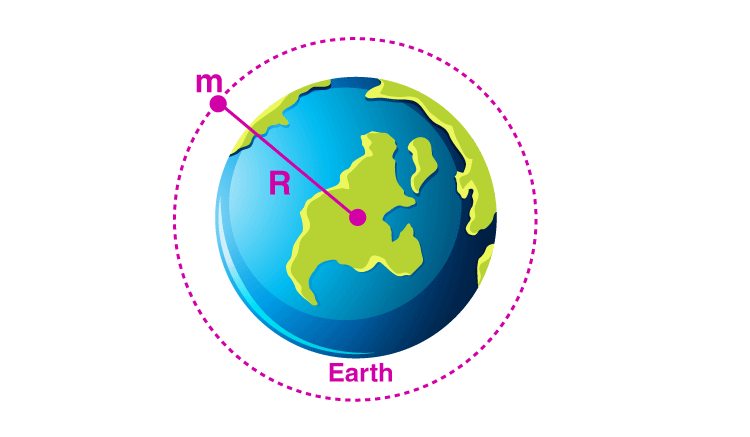a) K = 1/2mv2 = (1/2 m)(GM/R)

b) PE of satellite is U = -GMm/R = -2K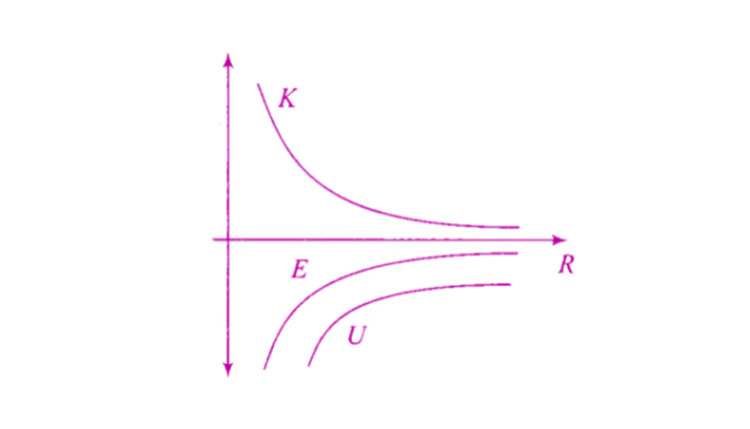c) Total energy of the satellite = -GMm/2R

8.31. Shown are several curves. Explain with reason, which ones amongst them can be possible trajectories traced by a projectile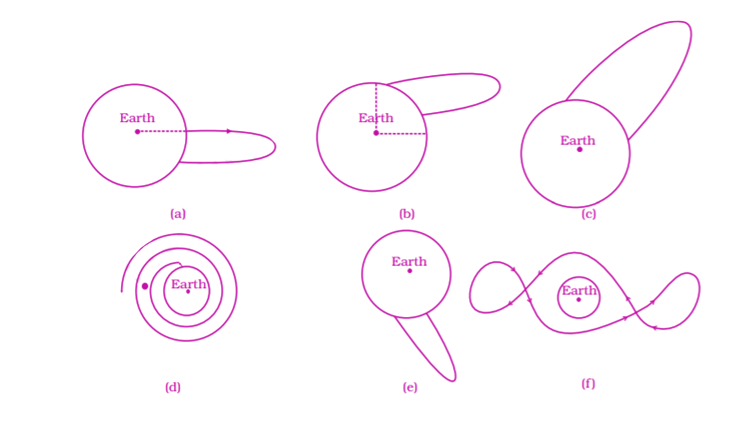The given options, option (c) show the focus of trajectory.

8.32. An object of mass m is raised from the surface of the earth to a height equal to the radius of the earth, that is, taken from a distance R to 2R from the centre of the earth. What is the gain in its potential energy?

The PE of the body on the earth = -GMm/R

When the body is close to the equator, the PE = -GMm/2R

Gain in PE = 1/2 mgR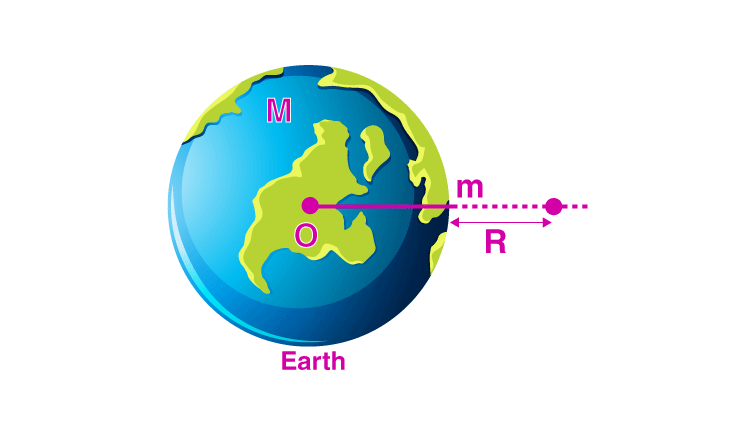8.33. A mass m is placed at P a distance h along the normal through the centre O of a thin circular ring of mass M and radius r. If the mass is removed further away such that OP becomes 2h, by what factor the force of gravitation will decrease, if h = r?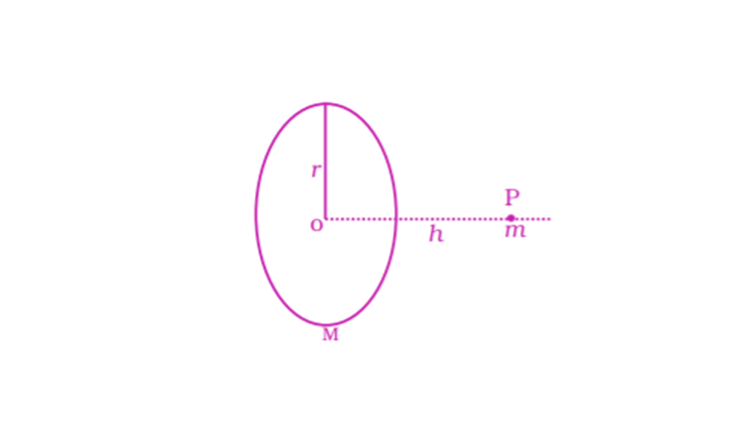Let r be the radius of the ring and m be the mass of the ring. Small element dM is considered as the mass, the gravitation force dF = G(dM)m/x2

Where x2 = r2 + h2

When the force is integrated, the distance between the m and the ring is 2h.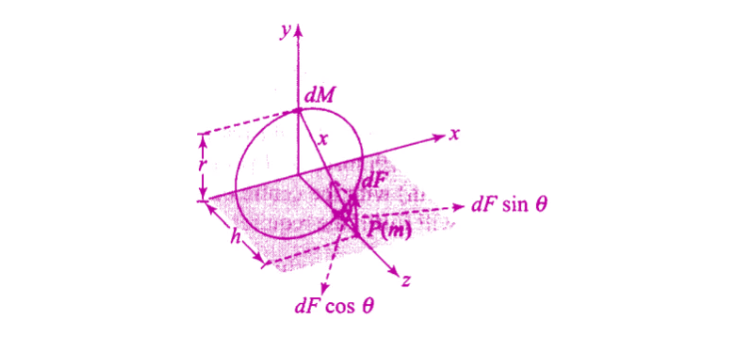8.34. A star like the sun has several bodies moving around it at different distances. Consider that all of them are moving in circular orbits. Let r be the distance of the body from the centre of the star and let its linear velocity be v, angular velocity ω, kinetic energy K, gravitational potential energy U, total energy E, and angular momentum l. As the radius r of the orbit increases, determine which of the above quantities increase and which ones decrease.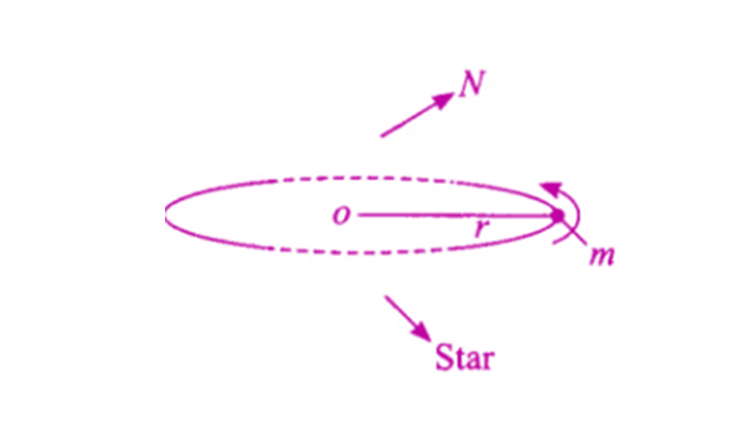8.35. Six-point masses of mass m each are at the vertices of a regular hexagon of side l. Calculate the force on any of the masses.

AE = AG + EG = AG + AG = 2AG = 2l cos 30o

AE = AC = √3l

Therefore,

Force on A due to B is Gm2/l2 along B to A

Force on A due to C is Gm2/3l2 along D to A

Force on A due to D is Gm2/4l2 along D to A

Force on A due to E is Gm2/3l2 along E to A

Therefore, total force = F = Gm2/l2 (1 + 1/√3 + 1/4) along DA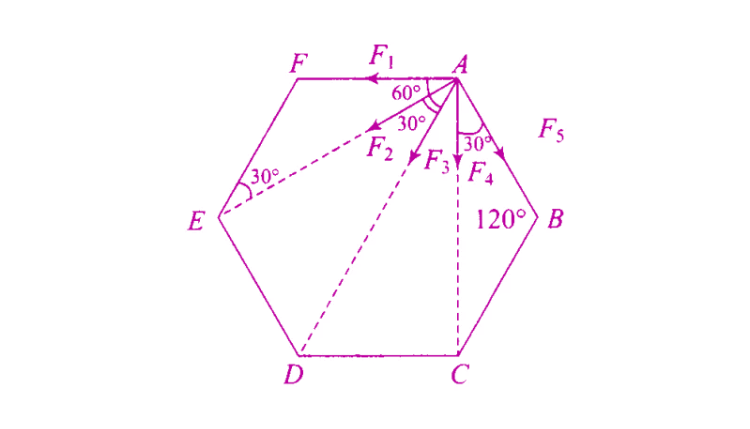8.36. A satellite is to be placed in equatorial geostationary orbit around the earth for communication

a) calculate height of such a satellite

b) find out the minimum number of satellites that are needed to cover entire earth so that at least one satellites is visible from any point on the equator

a) Mass of the earth, M = 6 1024 kg

Radius of the earth, R = 6.4 103 m

Time period = 24 36 102 s

G = 6.67 10-11 N.m2/kg2

Orbital radius = R + h

Using orbital velocity, we can calculate h = 35,940 km

b) The no.of satellites that are needed to cover the entire earth so that at least one satellite is visible from any point of the equator is 3.

8.37. Earth’s orbit is an ellipse with eccentricity 0.0167. Thus, the earth’s distance from the sun and speed as it moves around the sun varies from day to day. This means that the length of the solar day is not constant throughout the year. Assume that earth’s spin axis is normal to its orbital plane and find out the length of the shortest and the longest day. A day should be taken from noon to noon. Does this explain the variation of the length of the day during the year?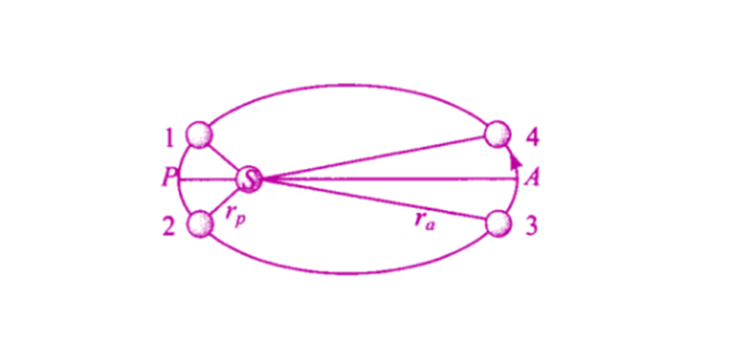Velocity of the earth at perigee = vp

Velocity of the earth at apogee = va

Angular velocity of the earth at perihelion = ꞷp

Angular velocity of the earth at aphelion = ꞷa

ꞷp/ꞷa = 1.0691

ꞷ is the mean angular speed = 1.0691

ꞷp/ꞷ = ꞷ/ꞷa = 1.034

Therefore, it can be said that this does not explain the actual variation of length of the day during the year.

8.38. A satellite is in an elliptic orbit around the earth with an aphelion of 6R and perihelion of 2R where R = 6400 km is the radius of the earth. Find eccentrically of the orbit. Find the velocity of the satellite at apogee and perigee. What should be done if this satellite has to be transferred to a circular orbit of radius 6R?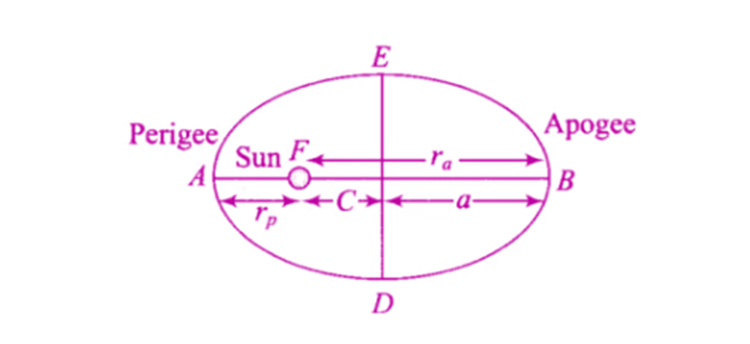Radius of perigee = rp = 2R

Radius of apogee = ra = 6R

rp = 2R

ra = 6R

rp = a(1-e) = 2R

ra = a(1+e) = 6R

From the above equation, e = 1/2

Since there is no external force on the system, from the law of conservation of angular momentum

L1 = L2

ma = mp = m = mass of the satellite

va/vp = rp/ra = 1/3

Therefore, vp = 3va

From conservation of energy we get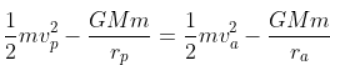va = 2.28 km/s

vc = 3.23 km/s

The velocity at the apogee is vc – va = 0.95 km/s

### Important topics of Chapter 8 Gravitation

1. Kepler’s laws
2. The universal law of gravitation
3. The gravitational constant
4. Acceleration due to the gravity of the earth
5. Acceleration due to gravity below and above the surface of the earth
6. Gravitational potential energy
7. Escape speed
8. Earth satellites
9. The energy of an orbiting satellite
10. Geostationary and polar satellites
11. Weightlessness.

### Why Opt for BYJU’s?

BYJU’S  have created some of the best NCERT exemplar with an aim to help students clear all their doubts and perform well in their examinations. The NCERT exemplar we are providing includes relevant exercises that have been solved by our highly qualified subject experts. Detailed explanations are also provided to give students a clear understanding and in-depth knowledge of the topic. The NCERT solutions that we are providing are the perfect study material.